## GATE 2016 [Set-2]

 Question 1

The man who is now Municipal Commissioner worked as _______________.

 A the security guard at a university B a security guard at the university C a security guard at university D the security guard at the university
Aptitude       Verbal
Question 1 Explanation:
Security guard is a general post, so we use the artical 'a' before the security.
And coming to university it is an organization.
So we use are 'the' before university.
 Question 2

Nobody knows how the Indian cricket team is going to cope with the difficult and seamer-friendly wickets in Australia.

Choose the option which is closest in meaning to the underlined phrase in the above sentence.

 A put up with B put in with C put down to D put up against
Aptitude       Verbal
Question 2 Explanation:
"Put up with" is means that "tolerate" which is the closest meaning of "cope with".
→ Cope with is doesn't means that put someone into competition with some other.
 Question 3

Find the odd one in the following group of words.

mock,   deride,   praise,   jeer
 A mock B deride C praise D jeer
Aptitude       Verbal
Question 3 Explanation:
Mock = Tease or laugh
Deride = express contempts for; ridicule
Jeer = Make rude and mocking remarks
→ These three are negative things
Praise = express warm approval
 Question 4

Pick the odd one from the following options.

 A CADBE B JHKIL C XVYWZ D ONPMQ
Aptitude       Numerical
Question 4 Explanation:
In all the given options except D, the 1st, 3rd, 5th alphabets are consecutive (increasing order) and 2nd and 4th are consecutive (increasing order).
But in D, 2nd and 4th are in decreasing order.
 Question 5

In a quadratic function, the value of the product of the roots (α, β) is 4. Find the value of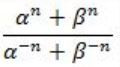A n4 B 4n C 22n-1 D 4n-1
Aptitude       Numerical
Question 5 Explanation:
Given,
Product of roots (α, β) = 4
⇒ αβ = 4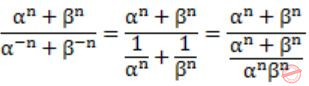(αβ)n = 4n
 Question 6

Among 150 faculty members in an institute, 55 are connected with each other through Facebook® and 85 are connected through WhatsApp®. 30 faculty members do not have Facebook® or WhatsApp® accounts. The number of faculty members connected only through Facebook® accounts is ______________.

 A 35 B 45 C 65 D 90
Aptitude       Numerical
Question 6 Explanation:
Total number of faculty = 150
Number of faculty members connected through Facebook = 55
Number of faculty members connected through Whatsapp = 85
Number of faculty members with Facebook (or) Whatsapp accounts = 30
Number of faculty members with either Facebook (or) Whatsapp accounts = 150 - 30 = 120
Number of faculty members with both Facebook and Whatsapp accounts = 85 + 55 - 120 = 20
Number of faculty members with only Facebook accounts = 55 - 20 = 35
 Question 7

Computers were invented for performing only high-end useful computations. However, it is no understatement that they have taken over our world today. The internet, for example, is ubiquitous. Many believe that the internet itself is an unintended consequence of the original invention. With the advent of mobile computing on our phones, a whole new dimension is now enabled. One is left wondering if all these developments are good or, more importantly, required.

Which of the statement(s) below is/are logically valid and can be inferred from the above paragraph?

(i) The author believes that computers are not good for us.
(ii) Mobile computers and the internet are both intended inventions.
 A (i) only B (ii) only C both (i) and (ii) D neither (i) nor (ii)
Aptitude       Verbal
Question 7 Explanation:
The author, has no where said that the computers are bad, authoring is talking about the way computers are being used today and the author questioned this way.
So, statement I does not follow.
→ "Many believes that the internet itself is unintended consequence of the original invention.
So, statement II does not follow, so Answer is Option D.
 Question 8

All hill-stations have a lake. Ooty has two lakes.

Which of the statement(s) below is/are logically valid and can be inferred from the above sentences?

(i) Ooty is not a hill-station.
(ii) No hill-station can have more than one lake.
 A (i) only B (ii) only C both (i) and (ii) D neither (i) nor (ii)
Aptitude       Verbal
Question 8 Explanation:
Given,
All hill-stations have a lake → (1)
Ooty has two lakes → (2)
From (1), it cannot be inferred that every place having a lake is a hill station.
⇒ (i) cannot be logically intended.
From (1), it cannot be inferred how many lakes will be there in a hill station.
⇒ (ii) cannot be logically inferred.
 Question 9

In a 2 × 4 rectangle grid shown below, each cell is a rectangle. How many rectangles can be observed in the grid?A 21 B 27 C 30 D 36
Aptitude       Numerical
Question 9 Explanation:
Number of rectangles in a grid with 'm' horizontal and 'n' vertical lines = 5C2 × 3C2 = 10 × 3 = 30
 Question 10Choose the correct expression for f(x) given in the graph.

 A f(x) = 1 - |x - 1| B f(x) = 1 + |x - 1| C f(x) = 2 - |x - 1| D f(x) = 2 + |x - 1|
Aptitude       Numerical
Question 10 Explanation:
From the graph,
x = -1 ⇒ f(x) = 0
x = 0 ⇒ f(x) = 1
We have check, which option satisfies both the conditions.
Only option (C) satisfies both of them.
 Question 11

Consider the following expressions:

(i) false
(ii) Q
(iii) true
(iv) P ∨ Q
(v) ¬Q ∨ P

The number of expressions given above that are logically implied by P ∧ (P ⇒ Q) is _________.

 A 4 B 5 C 6 D 7
Engineering-Mathematics       Propositional-Logic
Question 11 Explanation:
The expression is logically implied by P ∧ (P → Q) means
(P ∧ (P → Q))→ expression is a tautology. So we have to find
How many tautological formulas are there for the given inputs.
(P ∧ (P → Q)) → True is always tautology
(P ∧ (P → Q)) → False is not a tautology
(P ∧ (P → Q)) → Q is a tautology
(P ∧ (P → Q)) → ¬Q ∨ P is a tautology
(P ∧ (P → Q)) → P ∨ Q is a tautology
So there are 4 expressions logically implied by (P ∧ (P → Q))
 Question 12

Let f(x) be a polynomial and g(x) = f'(x) be its derivative. If the degree of (f(x) + f(-x)) is 10, then the degree of (g(x) - g(-x)) is __________.

 A 9 B 10 C 11 D 12
Engineering-Mathematics       Calculus
Question 12 Explanation:
If the degree of a polynomial is ‘n’ then the derivative of that function have (n – 1) degree.
It is given that f(x) + f(-x) degree is 10.
It means f(x) is a polynomial of degree 10.
Then obviously the degree of g(x) which is f’(x) will be 9.
 Question 13

The minimum number of colours that is sufﬁcient to vertex-colour any planar graph is ________.

 A 4 B 5 C 6 D 7
Engineering-Mathematics       Graph-Theory
Question 13 Explanation:
The 4-colour theorem of the planar graph describes that any planar can atmost be colored with 4 colors.
Here it is asked about the sufficient number of colors, so with the worst case of 4 colors we can color any planar graph.
 Question 14

Consider the systems, each consisting of m linear equations in n variables.

I. If m < n, then all such systems have a solution
II. If m > n, then none of these systems has a solution
III. If m = n, then there exists a system which has a solution

Which one of the following is CORRECT?

 A I, II and III are true B Only II and III are true C Only III is true D None of them is true
Engineering-Mathematics       Linear-Algebra
Question 14 Explanation:
i) If m In AX = B,
If R(A) ≠ R(A|B)
then there will be no solution.
ii) False, because if R(A) = R(A|B),
then there will be solution possible.
iii) True, if R(A) = R(A|B),
then there exists a solution.
 Question 15

Suppose that a shop has an equal number of LED bulbs of two different types. The probability of an LED bulb lasting more than 100 hours given that it is of Type 1 is 0.7, and given that it is of Type 2 is 0.4. The probability that an LED bulb chosen uniformly at random lasts more than 100 hours is _________.

 A 0.55 B 0.56 C 0.57 D 0.58
Engineering-Mathematics       Probability
Question 15 Explanation:The bulbs of Type 1, Type 2 are same in number.
So, the probability to choose a type is 1/2.
The probability to choose quadrant ‘A’ in diagram is
P(last more than 100 hours/ type1) = 1/2 × 0.7
P(last more than 100 hours/ type2) = 1/2 × 0.4
Total probability = 1/2 × 0.7 + 1/2 × 0.4 = 0.55
 Question 16

Suppose that the eigenvalues of matrix A are 1, 2, 4. The determinant of (A-1)T is _________.

 A 0.125 B 0.126 C 0.127 D 0.128
Engineering-Mathematics       Linear-Algebra
Question 16 Explanation:
Determinant of a matrix is product of the eigen values.
Given that eigen values are 1, 2, 4.
So, its determinant is 1*2*4 = 8
The determinant of (A-1)T = 1/ AT = 1/|A| = 1/8 = 0.125
 Question 17

Consider an eight-bit ripple-carry adder for computing the sum of A and B, where A and B are integers represented in 2’s complement form. If the decimal value of A is one, the decimal value of B that leads to the longest latency for the sum to stabilize is _________.

 A -1 B -2 C -3 D -4
Question 17 Explanation:
In the question, longest LATENCY means longest DELAY for the sum to get settle.
If we do 2's complement of 1 = 0000 0001, we get -1 = "1111 1111"So, if B = -1, every carry bit is 1.
 Question 18

Let, x1⊕x2⊕x3⊕x4 = 0 where x1, x2, x3, x4 are Boolean variables, and ⊕ is the XOR operator. Which one of the following must always be TRUE?

 A x1x2x3x4 = 0 B x1x3+x2 = 0 CD x1 + x2 + x3 + x4 = 0
Digital-Logic-Design       Boolean-Algebra
Question 18 Explanation:
Given expression is,
x1 ⊕ x2 ⊕ x3 ⊕ x4 = 0 -----(1)
A) x1x2x3 x4 = 0
Put x1 = 1, x2 = 1, x3 = 1, x4 = 1
The given equation will be zero, i.e.,
1 ⊕ 1 ⊕ 1 ⊕ 1 = 0
But,
x1x2x3 x4 ≠ 0
So, false.
B) x1x3 + x2 = 0
Put x1 = 1, x2 = 1, x3 = 0 , x4 = 0
The given equation will be zero, i.e.,
1 ⊕ 1 ⊕ 0 ⊕ 0 = 0
But,
x1x3 + x2 ≠ 0
So, false.
D) x1 + x2 + x3 + x4 = 0
Let x1=1, x2=1, x3=0, x4=0
The given equation will be zero, i.e.,
1 ⊕ 1 ⊕ 0 ⊕ 0 = 0
But,
x1 + x2 + x3 + x4 ≠ 0
So, false.
(i) True.
 Question 19

Let X be the number of distinct 16-bit integers in 2’s complement representation. Let Y be the number of distinct 16-bit integers in sign magnitude representation.

Then X-Y is _________.

 A 1 B 2 C 3 D 4
Digital-Logic-Design       Number-Systems
Question 19 Explanation:
X = 216
Since range is - 215 to 215 - 1
Y = 216 - 1
Here, +0 and -0 are represented separately.
X - Y = 216 - (216 - 1)
= 1
 Question 20

A processor has 40 distinct instructions and 24 general purpose registers. A 32-bit instruction word has an opcode, two register operands and an immediate operand. The number of bits available for the immediate operand ﬁeld is __________.

 A 16 bits B 17 bits C 18 bits D 19 bits
Computer-Organization       Machine-Instructions
Question 20 Explanation:
6 bits are needed for 40 distinct instructions (because, 25 < 40 < 26)
5 bits are needed for 24 general purpose registers (because, 24 < 24 < 25)
32-bit instruction word has an opcode (6 bit), two register operands (total 10 bits) and an immediate operand (x bits).
The number of bits available for the immediate operand field
⇒ x = 32 – (6 + 10) = 16 bits
 Question 21

Breadth First Search (BFS) is started on a binary tree beginning from the root vertex. There is a vertex t at a distance four from the root. If t is the n-th vertex in this BFS traversal, then the maximum possible value of n is _________.

 A 31 B 32 C 33 D 34
Data-Structures       BFS
Question 21 Explanation:
Given is a vertex t at a distance of 4 from the root.For distance 1, max possible value is (3).
Similarly, for distance 2, max value is (7).
So, maximum number of nodes = 2(h+1) - 1
For distance 4, 2(4+1) - 1 ⇒ 25 - 1 ⇒ 32 - 1 = 31
31 is the last node.So for distance 4, the maximum possible node will be 31 & minimum will be 16.
 Question 22

The value printed by the following program is __________.

```     void f(int* p, int m) {
m = m + 5;
*p = *p + m;
return;
}

void main() {
int i=5, j=10;
f(&i, j);
printf("%d", i+j);
}
```
 A 30 B 31 C 32 D 33
Programming       Programming
Question 22 Explanation:P is a pointer stores the address of i, & m is the formal parameter of j.
Now, m = m + 5;
*p = *p + m;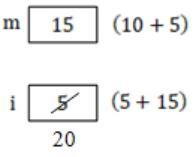Hence, i + j will be 20 + 10 = 30.
 Question 23

Assume that the algorithms considered here sort the input sequences in ascending order. If the input is already in ascending order, which of the following are TRUE?

I. Quicksort runs in Θ(n2) time
II. Bubblesort runs in Θ(n2) time
III. Mergesort runs in Θ(n) time
IV. Insertion sort runs in Θ(n) time
 A I and II only B I and III only C II and IV only D I and IV only
Algorithms       Sorting
Question 23 Explanation:
If input sequence is already sorted then the time complexity of Quick sort will take O(n2) and Bubble sort will take O(n) and Merge sort will takes O(nlogn) and insertion sort will takes O(n).
→ The recurrence relation for Quicksort, if elements are already sorted,
T(n) = T(n-1)+O(n) with the help of substitution method it will take O(n2).
→ The recurrence relation for Merge sort, is elements are already sorted,
T(n) = 2T(n/2) + O(n) with the help of substitution method it will take O(nlogn).
We can also use master's theorem [a=2, b=2, k=1, p=0] for above recurrence.
 Question 24

The Floyd-Warshall algorithm for all-pair shortest paths computation is based on

Algorithms       Floyd-Warshall-Algorithm
Question 24 Explanation:
→ All Pair shortest path algorithm is using Dynamic Programming technique.
It takes worst case time complexity is O(|V|3) and worst case space complexity is O(|V|2).
→ The Floyd–Warshall algorithm is an algorithm for finding shortest paths in a weighted graph with positive or negative edge weights (but with no negative cycles).
→ A single execution of the algorithm will find the lengths (summed weights) of the shortest paths between all pairs of vertices.
Although it does not return details of the paths themselves, it is possible to reconstruct the paths with simple modifications to the algorithm.
 Question 25

N items are stored in a sorted doubly linked list. For a delete operation, a pointer is provided to the record to be deleted. For a decrease-key operation, a pointer is provided to the record on which the operation is to be performed.

An algorithm performs the following operations on the list in this order: Θ(Ndelete,O(logN) insert, O(logN) ﬁnd, and Θ(N) decrease-key. What is the time complexity of all these operations put together?

 A O(log2 N) B O(N) C O(N2) D Θ(N2 logN)
Question 25 Explanation:
→ Delete needs O(1) time
→ Insert takes O(N) time
→ Find takes O(N) time
→ Decrease by takes O(N) time
Now number of each operation performed is given, so finally total complexity,
→ Delete = O(1) × O(N) = O(N)
→ Find = O(N) × O(log N) = O(N log N)
→ Insert = O(N) × O(log N) = O(N log N)
→ Decrease key = O(N) × O(N) = O(N2)
So, overall time complexity is, O(N2).
 Question 26

The number of states in the minimum sized DFA that accepts the language deﬁned by the regular expression

(0+1)*(0+1)(0+1)*
is_________.

 A 2 B 3 C 4 D 5
Theory-of-Computation       DFA
Question 26 Explanation:
The regular expression generates the min string “0” or “1” and then any number of 0’s and 1’s .
So, the DFA has two states.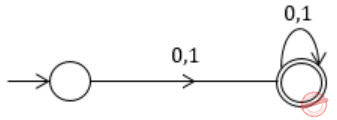Question 27

Language L1 is deﬁned by the grammar: S1 → aS1b|ε
Language L2 is deﬁned by the grammar: S2 → abS2

Consider the following statements:

P: L1 is regular
Q: L2 is regular

Which one of the following is TRUE?

 A Both P and Q are true B P is true and Q is false C P is false and Q is true D Both P and Q are false
Theory-of-Computation       Regular-Language
Question 27 Explanation:
The language L1 generated by the grammar contains equal number of a’s and b’s, but b’s comes after a’s.
So, in order to compare equality between a’s and b’s memory (stack) is required.
Hence, L1 is not regular.
Moreover, L1 = {an bn | n ≥ 0} which is DCFL.
The language L2 generated by grammar contains repetition of “ab” i.e. L2 = (ab)* which is clearly a regular language.
 Question 28

Consider the following types of languages: L1: Regular, L2: Context-free, L3 : Recursive, L4 : Recursively enumerable. Which of the following is/are TRUE?A I only B I and III only C I and IV only D I, II and III only
Theory-of-Computation       Closure-Property
Question 28 Explanation:
I.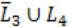L3 is recursive, sois also recursive (because recursive language closed under complementation), so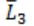is recursive enumerable.
L4 is recursive enumerable.
So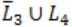is also recursive enumerable (closed under union).
II.L2 is context free, so L2 is recursive.
Since L2 is recursive. Sois recursive.
L3 is recursive.
Sois also recursive (closed under union)
III.L1 is regular, so L1* is also regular.
L2 is context free.
So, L1*∩L2 is also context free (closed under regular intersection).
IV.L1 is regular.
L2 is context free, somay or may not be context free (not closed under complement).
So,may or may not be context free.
 Question 29

Match the following:

```(P) Lexical analysis              (i) Leftmost derivation
(Q) Top down parsing             (ii) Type checking
(R) Semantic analysis           (iii) Regular expressions
(S) Runtime environments         (iv) Activation records
```
 A P ↔ i, Q ↔ ii, R ↔ iv, S ↔ iii B P ↔ iii, Q ↔ i, R ↔ ii, S ↔ iv C P ↔ ii, Q ↔ iii, R ↔ i, S ↔ iv D P ↔ iv, Q ↔ i, R ↔ ii, S ↔ iii
Compiler-Design       Compilers
Question 29 Explanation:
Regular expressions are used in lexical analysis.
Top down parsing has left most derivation of any string.
Type checking is done in semantic analysis.
Activation records are loaded into memory at runtime.
 Question 30

In which one of the following page replacement algorithms it is possible for the page fault rate to increase even when the number of allocated frames increases?

 A LRU (Least Recently Used) B OPT (Optimal Page Replacement) C MRU (Most Recently Used) D FIFO (First In First Out)
Operating-Systems       Page-Replacement-Algorithm
Question 30 Explanation:
In Belady’s anomaly is the phenomenon in which increasing the number of page frames results in an increase in the number of page faults for certain memory access patterns proves that it is possible to have more page faults when increasing the number of page frames while using the First in First Out (FIFO) page replacement algorithm.
In some situations, FIFO page replacement gives more page faults when increasing the number of page frames.
 Question 31

B+ Trees are considered BALANCED because

 A the lengths of the paths from the root to all leaf nodes are all equal. B the lengths of the paths from the root to all leaf nodes differ from each other by at most 1. C the number of children of any two non-leaf sibling nodes differ by at most 1. D the number of records in any two leaf nodes differ by at most 1.
Database-Management-System       B+-Trees
Question 31 Explanation:
In both B & B+ trees all the leaf nodes will be at same level.
 Question 32

Suppose a database schedule S involves transactions T1, ..., Tn. Construct the precedence graph of S with vertices representing the transactions and edges representing the conﬂicts. If S is serializable, which one of the following orderings of the vertices of the precedence graph is guaranteed to yield a serial schedule?

 A Topological order B Depth-ﬁrst order C Breadth-ﬁrst order D Ascending order of transaction indices
Database-Management-System       Transactions
Question 32 Explanation:
If a schedule is conflict serializable then no cycle in precedence graph should be present.
But BFS and DFS are also possible for cyclic graphs.
And topological sort is not possible for cyclic graph.
Moreover option (D) is also wrong because in a transaction with more indices might come before lower one.
 Question 33

Anarkali digitally signs a message and sends it to Salim. Veriﬁcation of the signature by Salim requires

 A A station stops to sense the channel once it starts transmitting a frame. B The purpose of the jamming signal is to pad the frames that are smaller than the minimum frame size. C A station continues to transmit the packet even after the collision is detected. D The exponential backoff mechanism reduces the probability of collision on retransmissions.
Computer-Networks       Security
Question 33 Explanation:
An Ethernet is the most popularly and widely used LAN network for data transmission.
It is a protocol of data link layer and it tells how the data can be formatted to transmit and how to place the data on network for transmission.
Now considering the Ethernet protocol we will discuss all the options one by one
I. In Ethernet the station does not required, stops to sense the channel prior frame transmission.
II. A Jamming signal used to inform all the other devices or stations about collision that has occurred, so that further data transmission is stopped.
III. No, stations sends jamming signal if collusion is detected
IV. To reduce the probability of collision on retransmissions an exponential back off mechanism is used.
This is only True.
 Question 34

In an Ethernet local area network, which one of the following statements is TRUE?

 A A station stops to sense the channel once it starts transmitting a frame. B The purpose of the jamming signal is to pad the frames that are smaller than the minimum frame size. C A station continues to transmit the packet even after the collision is detected. D The exponential backoff mechanism reduces the probability of collision on retransmissions.
Computer-Networks       Ethernet
Question 34 Explanation:
An Ethernet is the most popularly and widely used LAN network for data transmission.
It is a protocol of data link layer and it tells how the data can be formatted to transmit and how to place the data on network for transmission.
Now considering the Ethernet protocol we will discuss all the options one by one
I. In Ethernet the station does not required, stops to sense the channel prior frame transmission.
II. A Jamming signal used to inform all the other devices or stations about collision that has occurred, so that further data transmission is stopped.
III. No, stations sends jamming signal if collusion is detected
IV. To reduce the probability of collision on retransmissions an exponential back off mechanism is used.
This is only True.
 Question 35

Identify the correct sequence in which the following packets are transmitted on the network by a host when a browser requests a webpage from a remote server, assuming that the host has just been restarted.

 A HTTP GET request, DNS query, TCP SYN B DNS query, HTTP GET request, TCP SYN C DNS query, TCP SYN, HTTP GET request D TCP SYN, DNS query, HTTP GET request
Computer-Networks       TCP
Question 35 Explanation:
When a browser requests a web page from a remote server then that requests (URL address) will be mapped to IP address using DNS query, then TCP synchronization takes place after that HTTP verify whether it is existed in the web server or not.
 Question 36

A binary relation R on ℕ × ℕ is deﬁned as follows: (a,b)R(c,d) if a≤c or b≤d. Consider the following propositions:

P: R is reﬂexive
Q: R is transitive

Which one of the following statements is TRUE?

 A Both P and Q are true. B P is true and Q is false. C P is false and Q is true. D Both P and Q are false.
Engineering-Mathematics       Set-Theory
Question 36 Explanation:
For every a,b ∈ N,
a≤c ∨ b≤d
Let a≤a ∨ b≤b is true for all a,b ∈ N
So there exists (a,a) ∀ a∈N.
It is Reflexive relation.
Consider an example
c = (a,b)R(c,d) and (c,d)R(e,f) then (a,b)R(e,f)
This does not hold for any (a>e) or (b>f)
eg:
(2,2)R(1,2) as 2≤2
(1,2)R(1,1) as 1≤1
but (2,2) R (1,1) is False
So, Not transitive.
 Question 37

Which one of the following well-formed formulae in predicate calculus is NOT valid?

 A (∀x p(x) ⇒ ∀x q(x)) ⇒ (∃x ¬p(x) ∨ ∀x q(x)) B (∃x p(x) ∨ ∃x q(x)) ⇒ ∃x (p(x) ∨ q(x)) C ∃x (p(x) ∧ q(x)) ⇒ (∃x p(x) ∧ ∃x q(x)) D ∀x (p(x) ∨ q(x)) ⇒ (∀x p(x) ∨ ∀x q(x))
Engineering-Mathematics       Propositional-Logic
Question 37 Explanation:
For the formulae to be valid there should not be implication like T → F.
But in option (D), we can generate T → F.
Hence, not valid.
 Question 38

Consider a set U of 23 different compounds in a Chemistry lab. There is a subset S of U of 9 compounds, each of which reacts with exactly 3 compounds of U. Consider the following statements:

I. Each compound in U\S reacts with an odd number of compounds.
II. At least one compound in U\S reacts with an odd number of compounds.
III. Each compound in U\S reacts with an even number of compounds.

Which one of the above statements is ALWAYS TRUE?

 A Only I B Only II C Only III D None
Engineering-Mathematics       Set-Theory
Question 38 Explanation:
There are set of ‘23’ different compounds.
U = 23
∃S ∋ (S⊂U)
Each component in ‘S’ reacts with exactly ‘3’ compounds of U,If a component ‘a’ reacts with ‘b’, then it is obvious that ‘b’ also reacts with ‘a’.
It’s a kind of symmetric relation.>br> If we connect the react able compounds, it will be an undirected graph.
The sum of degree of vertices = 9 × 3 = 27
But, in the graph of ‘23’ vertices the sum of degree of vertices should be even because(di = degree of vertex i.e., = no. of edges)
But ‘27’ is not an even number.
To make it an even, one odd number should be added.
So, there exists atleast one compound in U/S reacts with an odd number of compounds.
 Question 39

The value of the expression 1399(mod 17), in the range 0 to 16, is ________.

 A 4 B 5 C 6 D 7
Engineering-Mathematics       Modular-Arithmetic
Question 39 Explanation:
Fermat’s theorem,
a(p-1) ≡ 1 mod p (p is prime)
From given question,
p = 17
a(17-1) ≡ 1 mod 17
a16 ≡ 1 mod 17
1316 ≡ 1 mod 17
Given:
1399 mod 17133 mod 17
2197 mod 17
4
 Question 40

Suppose the functions F and G can be computed in 5 and 3 nanoseconds by functional units UF and UG, respectively. Given two instances of UF and two instances of UG, it is required to implement the computation F(G(Xi)) for 1 ≤ i ≤ 10. Ignoring all other delays, the minimum time required to complete this computation is _________ nanoseconds.

 A 28 B 29 C 30 D 31
Computer-Organization       Datapath
Question 40 Explanation:
We have to do 10 calculations of U and G as the loop runs from i = 1 to 10.
Since we have 2 instances of UF and UG, each unit need to be run 5 times to complete the execution.
Suppose computation starts at time 0, which means G starts at 0 and F starts at 3rd second since F is dependent on G and G finishes computing first element at 3rd second.
Computation of F ten times using two UF units can be done in 5*10/2 = 25ns.
For the start UF needs to wait for UG output for 3ns and rest all are pipelined and hence no more wait.
So, answer is 3 + 25 = 28ns
We can see the timing diagram below:Question 41

Consider a processor with 64 registers and an instruction set of size twelve. Each instruction has ﬁve distinct ﬁelds, namely, opcode, two source register identiﬁers, one destination register r identiﬁer, and a twelve-bit immediate value. Each instruction must be stored in memory in a byte-aligned fashion. If a program has 100 instructions, the amount of memory (in bytes) consumed by the program text is _________.

 A 500 bytes B 501 bytes C 502 bytes D 503 bytes
Computer-Organization       Machine-Instructions
Question 41 Explanation:
One instruction is divided into five parts,
(i) The opcode- As we have instruction set of size 12, an instruction opcode can be identified by 4 bits, as 24 = 16 and we cannot go any less.
(ii) & (iii) Two source register identifiers- As there are total 64 registers, they can be identified by 6 bits. As they are two i.e. 6 bit + 6 bit.
iv) One destination register identifier- Again it will be 6 bits.
v) A twelve bit immediate value- 12 bit.
4 + 6 + 6 + 6 + 12 = 34 bit = 34/8 byte = 4.25 bytes.
Due to byte alignment total bytes per instruction = 5 bytes.
As there are 100 instructions, total size = 5*100 = 500 Bytes.
 Question 42

The width of the physical address on a machine is 40 bits. The width of the tag ﬁeld in a 512 KB 8-way set associative cache is _________ bits.

 A 24 B 25 C 26 D 27
Computer-Organization       Cache
Question 42 Explanation:
Given that it is a set associative cache, so the physical address is divided as following:
(Tag bits + bits for set no. + Bits for block offset)
In question block size has not been given, so we can assume block size 2x Bytes.
The cache is of size 512KB, so number of blocks in the cache = 219/2x = 219-x
It is 8-way set associative cache so there will be 8 blocks in each set.
So number of sets = (219 − x)/8 = 216 − x
So number of bits for sets = 16−x
Let number of bits for Tag = T
Since we assumed block size is 2x Bytes, number of bits for block offset is x.
So, T + (16−x) + x = 40
T + 16 = 40
T = 24
 Question 43

Consider a 3 GHz (gigahertz) processor with a three-stage pipeline and stage latencies τ1, τ2, τ3 and such that τ = 3τ2/4 = 2τ3. If the longest pipeline stage is split into two pipeline stages of equal latency, the new frequency is _________ GHz, ignoring delays in the pipeline registers.

 A 4 B 5 C 6 D 7
Computer-Organization       Pipelining
Question 43 Explanation:
Given 3 stage pipeline, with 3 GHz processor.
Given, τ1 = 3 τ2/4 = 2 τ3
Put τ1 = 6t, we get τ2 = 8t, τ3 = 3t
Now largest stage time is 8t.
So, frequency is 1/8t
⇒ 1/8t = 3 GHz
⇒ 1/t = 24 GHz
From the given 3 stages, τ 1 = 6t, τ 2 = 8t and τ 3 = 3t
So, τ 2 > τ1 > τ3.
The longest stage is τ2 = 8t and we will split that into two stages of 4t & 4t.
New processor has 4 stages - 6t, 4t, 4t, 3t.
Now largest stage time is 6t.
So, new frequency is = 1/6t
We can substitute 24 in place of 1/t, which gives the new frequency as 24/6 = 4 GHz
 Question 44

A complete binary min-heap is made by including each integer in [1, 1023] exactly once. The depth of a node in the heap is the length of the path from the root of the heap to that node. Thus, the root is at depth 0. The maximum depth at which integer 9 can appear is _________.

 A 8 B 9 C 10 D 11
Data-Structures       Heap-Tree
Question 44 Explanation:This is not possible because it violates a property of complete binary tree.
We have total [0, 1023] elements. It means that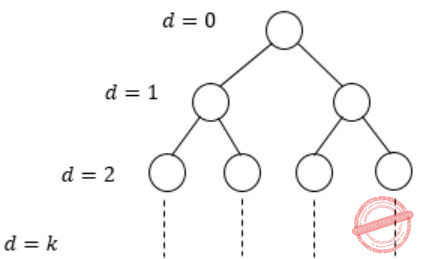∴ 20 + 21 + 22 + ⋯ + 2k = 1024
Add if 1(2(k+1)-1)/(2-1) [using formula for sum of k terms k+1 in G.P]
= 2(k+1) - 1 = 1024 - 1 = 1023
∴ The level ‘9’ at the depth of 8.
Actually we have 1023 elements, we can achieve a complete binary min heap of depth 9, which would cover all 1023 elements, but the max depth of node 9 can be only be 8.
 Question 45

The following function computes XY for positive integers X and Y.

```       int exp (int X, int Y) {
int res = 1, a = X, b = Y;

while ( b != 0 ){
if ( b%2 == 0) { a = a*a; b = b/2; }
else           { res = res*a; b = b-1; }
}
return res;

}
```

Which one of the following conditions is TRUE before every iteration of the loop?

 A XY = ab B (res * a)Y = (res * X)b C XY = res * ab D XY = (res * a)b
Programming       Programming
Question 45 Explanation:
int exp (int X, int Y)
{
int res = 1, a = X, b = Y;
while (b != 0)
{
if (b%2 == 0)
{
a = a*a;
b = b/2;
}
else
{
res = res*a;
b = b – 1;
}
}
return res;
}
From that explanation part you can understand the exponent operation, but to check the conditions, first while iteration is enough.
x = 2, y = 3, res = 2, a = 2, b = 2.
Only (C) satisfies these values.
xy = res * ab
23 = 2 * 22 = 8
Explanation:
Will compute for smaller values.
Let X = 2, Y = 3, res = 1
while (3 != 0)
{
if(3%2 == 0) - False
else
{
res = 1*2 = 2;
b = 3 – 1 = 2;
}
For options elimination, consider
return res = 2 (but it is out of while loop so repeat while)
__________
while (2 != 0)
{
if (2%2 == 0) - True
{
a = 2*2 = 4
b = 2/2 = 1
}
__________
repeat while
while (1 != 0)
{
if (1%2 == 0) - False
else
{
res = 2 * 4 = 8
b = 1 – 1 = 0
}
__________
while (0 != 0) - False
return res = 8 (23)
 Question 46

Consider the following New-order strategy for traversing a binary tree:

• Visit the root;
• Visit the right subtree using New-order;
• Visit the left subtree using New-order;

The New-order traversal of the expression tree corresponding to the reverse polish expression 3 4 * 5 - 2 ˆ 6 7 * 1 + - is given by:

 A + - 1 6 7 * 2 ˆ 5 - 3 4 * B - + 1 * 6 7 ˆ 2 - 5 * 3 4 C - + 1 * 7 6 ˆ 2 - 5 * 4 3 D 1 7 6 * + 2 5 4 3 * - ˆ -
Data-Structures       Binary-Trees
Question 46 Explanation:
New Order strategy: Root, Right, Left.
Given Reverse Polish Notation as:
3 4 * 5 - 2 ^ 6 7 * 1 + -
We know Reverse Polish Notation takes Left, Right, Root.So the expression tree looks likeFrom the tree, we can write the New Order traversal as: Root, Right, Left.
- + 1 * 7 6 ^ 2 - 5 * 4 3
 Question 47

Consider the following program:

```    int f(int *p, int n)
{
if (n <= 1) return 0;
else return max (f(p+1,n-1),p-p);
}

int main()
{
int a[] = {3,5,2,6,4};
printf("%d", f(a,5));
}
```
Note: max(x,y) returns the maximum of x and y.

The value printed by this program is __________.

 A 3 B 4 C 5 D 6
Programming       Programming
Question 47 Explanation:
Givenf(a, 5) ⇒ f(100, 5)Question 48

Let A1, A2, A3 and A4 be four matrices of dimensions 10 × 5, 5 × 20, 20 × 10, and 10 × 5, respectively. The minimum number of scalar multiplications required to ﬁnd the product A1A2A3A4 using the basic matrix multiplication method is _________.

 A 1500 B 1501 C 1502 D 1503
Algorithms       Matrix-Chain-Multiplication
Question 48 Explanation:
→ The minimum number of scalar multiplications required is 1500.
The optimal parenthesized sequence is A1((A2A3)A4) out of many possibilities, the possibilities are
1. ((A1A2)A3)A4
2. ((A1(A2A3))A4)
3. (A1A2)(A3A4)
4. A1((A2A3)A4)
5. A1(A2(A3A4))
→ A1((A2A3)A4) = (5 x 20 x 10) + (5 x 10 x 5) + (10 x 5 x 5) = 1000 + 250 + 250 = 1500
 Question 49

The given diagram shows the ﬂowchart for a recursive function A(n). Assume that all statements, except for the recursive calls, have O(1) time complexity. If the worst case time complexity of this function is O(nα), then the least possible value (accurate upto two decimal  positions) of α is _________.A 2.32193 B 2.32193 C 2.32193 D 2.32193
Algorithms       Time-Complexity
Question 49 Explanation:
This type of problem, we have to consider worst case time complexity, it mean that all possibilities.
According to flow chart total 5 worst case possibilities of function calls.The remaining function calls/return statements will execute only constant amount of time.
So, total function calls 5.
The Recurrence will be
A(n) = 5A(n/2) + O(1)
Apply Master’s theorem,
A=5, b=2, k=0, p=0
a > bk case
A(n) = n(logba ) = n(log25) = n2.3219280
∴α = 2.3219280
 Question 50

The number of ways in which the numbers 1, 2, 3, 4, 5, 6, 7 can be inserted in an empty binary search tree, such that the resulting tree has height 6, is _________.

Note: The height of a tree with a single node is 0.
 A 64 B 65 C 66 D 67
Data-Structures       Trees
Question 50 Explanation:
To get the tree of height 6, every level should contain only 1 node.
So to get such tree at each level we should have either maximum or minimum element from remaining numbers till that level. So no. of binary search tree possible is,
1st level - 2 (maximum or minimum)

2nd level - 2

3rd level - 2

4th level - 2

5th level - 2

6th level - 2

7th level - 2
= 2 × 2 × 2 × 2 × 2 × 2 × 1
= 26
= 64
 Question 51

In an adjacency list representation of an undirected simple graph G = (V,E), each edge (u,v) has two adjacency list entries: [v] in the adjacency list of u, and [u] in the adjacency list of v. These are called twins of each other. A twin pointer is a pointer from an adjacency list entry to its twin. If |E| = m and |V| = n, and the memory size is not a constraint, what is the time complexity of the most efﬁcient algorithm to set the twin pointer in each entry in each adjacency list?

 A Θ(n2) B Θ(n+m) C Θ(m2) D Θ(n4)
Data-Structures       Graphs
Question 51 Explanation:
Applying BFS on Undirected graph give you twin pointer.
Visit every vertex level-wise for every vertex fill adjacent vertex in the adjacency list. BFS take O(m+n) time.
Note:
Twin Pointers can be setup by keeping track of parent node in BFS or DFS of graph.
 Question 52

Consider the following two statements:

I. If all states of an NFA are accepting states then the language accepted by the NFA is Σ*.
II. There exists a regular language A such that for all languages B, A∩B is regular.

Which one of the following is CORRECT?

 A Only I is true B Only II is true C Both I and II are true D Both I and II are false
Theory-of-Computation       Regular-and-Finite-Automata
Question 52 Explanation:
Statement I is false:
The reason is NFA doesn’t have dead state, so even though all states are final state in NFA, the NFA will reject some strings.
For ex:
Consider L = a*b*
The NFA would be: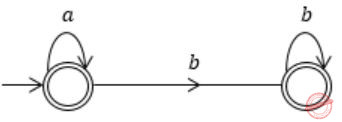Even though all states are final states in above NFA, but it doesn’t accept string “aba”.
Hence its language can’t be ∑*.
Statement II is true:
Since A= Φ is a regular language and its intersection with any language B will be Φ (which is regular).
 Question 53

Consider the following languages:

L1 = {an bm cn+m : m,n ≥ 1}
L2 = {an bn c2n : n ≥ 1}

Which one of the following is TRUE?

 A Both L1 and L2 are context-free. B L1 is context-free while L2 is not context-free. C L2 is context-free while L1 is not context-free. D Neither L1 nor L2 is context-free.
Theory-of-Computation       Context-Free-Language
Question 53 Explanation:
L1 can be recognized by PDA, we have to push a’s and b’s in stack and when c’s comes then pop every symbol from stack for each c’s.
At the end if input and stack is empty then accept.
Hence, it is CFL.
But L2 can’t be recognized by PDA, i.e. by using single stack.
The reason is, it has two comparison at a time,
1st comparison:
number of a’s = number of b’s
2nd comparison:
number of c’s must be two times number of a’s (or b’s)
It is CSL.
 Question 54

Consider the following languages.

L1 = {〈M〉|M takes at least 2016 steps on some input},
L2 = {〈M〉│M takes at least 2016 steps on all inputs} and
L3 = {〈M〉|M accepts ε},

where for each Turing machine M, 〈M〉 denotes a speciﬁc encoding of M. Which one of the following is TRUE?

 A L1 is recursive and L2, L3 are not recursive B L2 is recursive and L1, L3 are not recursive C L1, L2 are recursive and L3 is not recursive D L1, L2, L3 are recursive
Theory-of-Computation       Turing Machine
Question 54 Explanation:
L1 is recursive:
Since counting any number of steps can be always decided.
We can simulate TM (M) whether it takes more than 2016 steps on some input string, which has length upto 2016.
If it happens then reached to accepting (YES) state else reject (NO).
L2 is recursive:
Similarly, we can simulate TM (M) whether it takes more than 2016 steps on each input string which has length upto 2016.
If it happens then reached to accepting (YES) state else reject (NO).
L3 is not recursive:
If L3 is recursive then we must have a Turing machine for L3, which accept epsilon and reject all strings and always HALT.
Since Halting of Turing machine can’t be guaranteed in all the case.
Hence this language is not recursive.
 Question 55

Which one of the following grammars is free from left recursion?

 A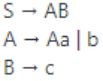B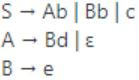C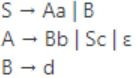DCompiler-Design       Left-Recursive-Grammar
Question 55 Explanation:
The grammar in option A has direct left recursion because of production (A→Aa).
The grammar in option C has indirect left recursion because of production, (S→Aa and A→Sc).
The grammar in option D also has indirect left recursion because of production, (A→Bd and B→Ae).
 Question 56

A student wrote two context-free grammars G1 and G2 for generating a single C-like array declaration. The dimension of the array is at least one. For example,

int a;
The grammars use D as the start symbol, and use six terminal symbols int; id[] num.
```Grammar G1               Grammar G2
D → intL;                 D → intL;
L → id[E                  L → idE
E → num]                  E → E[num]
E → num][E                E → [num]
```
Which of the grammars correctly generate the declaration mentioned above?

 A Both G1 and G2 B Only G1 C Only G2 D Neither G1 nor G2
Theory-of-Computation       Membership-Function
Question 56 Explanation:
Both grammars G1 and G2 generate C-array like declaration:Question 57

Consider the following processes, with the arrival time and the length of the CPU burst given in milliseconds. The scheduling algorithm used is preemptive shortest remaining-time ﬁrst.The average turn around time of these processes is _________ milliseconds.

 A 8.25 B 8.26 C 8.27 D 8.28
Operating-Systems       Process-Scheduling
Question 57 Explanation:
Here the scheduling algorithm used is preemptive shortest remaining-time first.
To answer the question we need to design the gantt chart: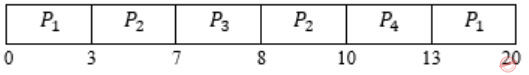In this algorithm, the processes will be scheduled on the CPU which will be having least remaining burst time.
Turnaround Time (TAT) = Completion Time (CT) - Arrival Time (AT)
TAT for P1 = 20 - 0 = 20,
TAT for P2 = 10 - 3 = 7,
TAT for P3 = 8 - 7 = 1,
TAT for P4 = 13 - 8 = 5.
Total TAT = 20 + 7 + 1 + 5 = 33 / 4 = 8.25 (Avg. TAT)
 Question 58

Consider the following two-process synchronization solution.

```Process 0                                     Process 1
---------                                     ---------
Entry: loop while (turn == 1);                Entry: loop while (turn == 0);
(critical section)                            (critical section)
Exit: turn = 1;                               Exit: turn = 0;
```

The shared variable turn is initialized to zero. Which one of the following is TRUE?

 A This is a correct two-process synchronization solution. B This solution violates mutual exclusion requirement. C This solution violates progress requirement. D This solution violates bounded wait requirement.
Operating-Systems       Process-Synchronization
Question 58 Explanation:
B) Mutual exclusion is satisfied because the value of turn variable cannot be 0 and 1 at the same time.
So False.
C) Progress means if one process does not want to enter the critical section then it should not stop other process to enter the critical section.
But we can see that if process 0 will not enter the critical section then value of turn will not become 1 and process 1 will not be able to enter critical section.
So progress not satisfied. True.
D) Bounded waiting solution as there is a strict alteration.
So, False.
 Question 59

Consider a non-negative counting semaphore S. The operation P(S) decrements S, and V(S) increments S. During an execution, 20 P(S) operations and 12 V(S) operations are issued in some order. The largest initial value of S for which at least one P(S) operation will remain blocked is _________.

 A 7 B 8 C 9 D 10
Operating-Systems       Process-Synchronization
Question 59 Explanation:
We can assume the largest initial value of S for which at least one P(S) operation → X
P(S) operation remain in blocked state therefore it will -1.
The negative value of the counting semaphore indicates the number of processes in suspended list (or blocked).
Take any sequence of 20P and 12V operations, at least one process will always remain blocked.
So, X - 20 + 12 = -1
Here P(S) = 20 and V(S) = 12
X = 7
 Question 60

A ﬁle system uses an in-memory cache to cache disk blocks. The miss rate of the cache is shown in the ﬁgure. The latency to read a block from the cache is 1 ms and to read a block from the disk is 10 ms. Assume that the cost of checking whether a block exists in the cache is negligible. Available cache sizes are in multiples of 10 MB.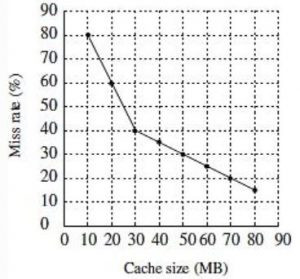The smallest cache size required to ensure an average read latency of less than 6 ms is _______ MB.

 A 30 B 31 C 32 D 33
Computer-Organization       Cache
Question 60 Explanation:
Here it is not given whether the memory is hierarchical or simultaneous.
So we consider it as hierarchical memory.
But it is given that “assume that the cost of checking whether a block exists in the cache is negligible”, which means don't consider the checking time in the cache when there is a miss.
So formula for average access time becomes h1t1 + (1-h1)(t2) which is same as for simultaneous access.
Though the memory is hierarchical because of the statement given in the question we ignored the cache access time when there is a miss and effectively the calculation became like simultaneous access.
The average access time or read latency = h1t1 + (1-h1)t2.
It is given that the average read latency has to be less than 6ms.
So, h1t1 + (1-h1)t2 < 6
From the given information t1 = 1ms, t2 = 10ms
h1*1+(1-h1)10 < 6
10-9h1 < 6
-9h1 < - 4
-h1 < - 4/9
-h1 < -0.444
Since in the given graph we have miss rate information and 1-h1 gives the miss rate, so we add 1 on both sides of the above inequality.
1-h1 < 1-0.444
1-h1 < 0.555
So for the average read latency to be less than 6 ms the miss rate hsa to be less than 55.5%.
From the given graph the closest value of miss rate less than 55.5 is 40%.
For 40% miss rate the corresponding cache size is 30MB.
 Question 61

Consider the following database schedule with two transactions, T1 and T2.

S = r2(X); r1(X); r2(Y); w1(X); r1(Y); w2(X); a1; a2

where ri(Z) denotes a read operation by transaction Ti on a variable Z, wi(Z) denotes a write operation by Ti on a variable Z and ai denotes an abort by transaction Ti.

Which one of the following statements about the above schedule is TRUE?

 A S is non-recoverable B S is recoverable, but has a cascading abort C S does not have a cascading abort D S is strict
Database-Management-System       Transactions
Question 61 Explanation:
Given schedule is,Now let's check statements one by one,
A) False, because there is no dirty read. So, it is recoverable.
B) False, because there is to dirty read. So, no cascading aborts.
C) True.
D) False, because there is Transaction T2 which written the value of x which is written by T1 before T1 has aborted. So, not strict.
 Question 62

Consider the following database table named water_schemes :The number of tuples returned by the following SQL query is _________.

```with total(name, capacity) as
select district_name, sum(capacity)
from water_schemes
group by district_name
with total_avg(capacity) as
select avg(capacity)
from total
select name
from total, total_avg
where total.capacity ≥ total_avg.capacity
```
 A 2 B 3 C 4 D 5
Database-Management-System       SQL
Question 62 Explanation:
• The SQL WITH clause allows you to give a sub-query block a name (a process also called sub-query refactoring), which can be referenced in several places within the main SQL query.
The name assigned to the sub-query is treated as though it was an inline view or table.
• First group by district name is performed and total capacities are obtained as following:• Then average capacity is computed,
Average Capacity = (20 + 40 + 30 + 10)/4
= 100/4
= 25
• Finally, 3rd query will be executed and it's tuples will be considered as output, where name of district and its total capacity should be more than or equal to 25.
• Then average capacity is computed,
Average Capacity = (20 + 40 + 30 + 10)/4
= 100/4
= 25
• Finally, 3rd query will be executed and it's tuples will be considered as output, where name of district and its total capacity should be more than or equal to 25.Question 63

A network has a data transmission bandwidth of 20 × 106 bits per second. It uses CSMA/CD in the MAC layer. The maximum signal propagation time from one node to another node is 40 microseconds. The minimum size of a frame in the network is _________ bytes.

 A 200 B 201 C 202 D 203
Computer-Networks       CSMA/CD
Question 63 Explanation:
For frame size to be minimum, its transmission time should be equal to twice of one way propagation delay. i.e, Tt = 2 × TP
Given,
Bandwidth (B) = 20 × 106 bps
TP = 40 μs ⇒ 40 × 10- 6 sec
Suppose minimum frame size is L.
Tt = 2 × TP ⇒ L / B = 2 × TP
⇒ L = 2 × TP × B = 2 × 40 × 10-6 × 20 × 106 = 1600 bits ⇒ 200 bytes
Therefore, L = 200 bytes
 Question 64

For the IEEE 802.11 MAC protocol for wireless communication, which of the following statements is/are TRUE?

I. At least three non-overlapping channels are available for transmissions.
II. The RTS-CTS mechanism is used for collision detection.
III. Unicast frames are ACKed.
 A All I, II and III B I and III only C II and III only D II only
Computer-Networks       IEEE802.11
Question 64 Explanation:
802.11 MAC = Wifi
I. This is true, maximum 3 overlapping channels are possible in Wifi.
II. The RTS (Request To Send) and CTS(Clear To Send) are control frames which is used for collision avoidance, not in collision detection, (so, II is False)
III. Every frame in Wifi is Acked, because Wifi stations do not use collusion detection. (True)
 Question 65

Consider a 128 × 103 bits/ second satellite communication link with one way propagation delay of 150 milliseconds. Selective retransmission (repeat) protocol is used on this link to send data with a frame size of 1 kilobyte. Neglect the transmission time of acknowledgement. The minimum number of bits required for the sequence number ﬁeld to achieve 100% utilization is __________.

 A 4 B 5 C 6 D 7
Computer-Networks       SR-Protocol
Question 65 Explanation:
To achieve 100% efficiency, the number of frames that we should send N = 1 + 2 * a
a = Tp / Tt where Tp is propagation delay, and Tt is transmission delay.
Given, B = 128 kbps, Tp = 150 msec,
L = 1 KB = 1 * 8 * 210 bits
Tt = L / B ⇒ 1 * 8 * 210 bits / 128 * 103 bps ⇒ 0.064 sec = 64 msec
So, a = 150 msec / 64 msec = 2.343
Efficiency (η) = 100 % ⇒ 1 = N/ 1 + 2 * a
So, N = 1 + 2 * a ⇒ 1 + 2 * 2.343 = 5.686
No. of sequence numbers requires in SR is 2*N = 2 *5.686 = 11.375
Minimum No. of bits required in the sequence number = [ log2 (11.375) ] = 4
There are 65 questions to complete.